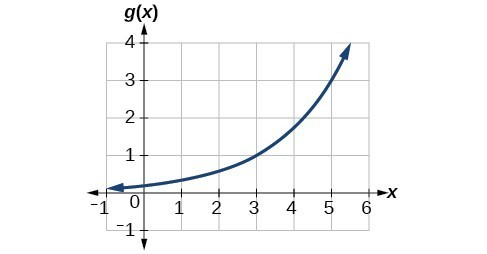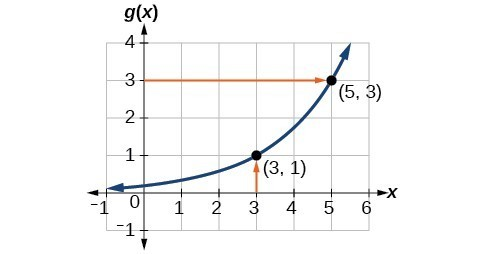## Find or evaluate the inverse of a function

Once we have a one-to-one function, we can evaluate its inverse at specific inverse function inputs or construct a complete representation of the inverse function in many cases.

## Inverting Tabular Functions

Suppose we want to find the inverse of a function represented in table form. Remember that the domain of a function is the range of the inverse and the range of the function is the domain of the inverse. So we need to interchange the domain and range.

Each row (or column) of inputs becomes the row (or column) of outputs for the inverse function. Similarly, each row (or column) of outputs becomes the row (or column) of inputs for the inverse function.

### Example 5: Interpreting the Inverse of a Tabular Function

A function $f\left(t\right)$ is given below, showing distance in miles that a car has traveled in $t$ minutes. Find and interpret ${f}^{-1}\left(70\right)$.

 $t\text{ (minutes)}$ 30 50 70 90 $f\left(t\right)\text{ (miles)}$ 20 40 60 70

### Solution

The inverse function takes an output of $f$ and returns an input for $f$. So in the expression ${f}^{-1}\left(70\right)$, 70 is an output value of the original function, representing 70 miles. The inverse will return the corresponding input of the original function $f$, 90 minutes, so ${f}^{-1}\left(70\right)=90$. The interpretation of this is that, to drive 70 miles, it took 90 minutes.

Alternatively, recall that the definition of the inverse was that if $f\left(a\right)=b$, then ${f}^{-1}\left(b\right)=a$. By this definition, if we are given ${f}^{-1}\left(70\right)=a$, then we are looking for a value $a$ so that $f\left(a\right)=70$. In this case, we are looking for a $t$ so that $f\left(t\right)=70$, which is when $t=90$.

### Try It 5

Using the table below, find and interpret (a) $\text{ }f\left(60\right)$, and (b) $\text{ }{f}^{-1}\left(60\right)$.

 $t\text{ (minutes)}$ 30 50 60 70 90 $f\left(t\right)\text{ (miles)}$ 20 40 50 60 70

Solution

## Evaluating the Inverse of a Function, Given a Graph of the Original Function

We saw in Functions and Function Notation that the domain of a function can be read by observing the horizontal extent of its graph. We find the domain of the inverse function by observing the vertical extent of the graph of the original function, because this corresponds to the horizontal extent of the inverse function. Similarly, we find the range of the inverse function by observing the horizontal extent of the graph of the original function, as this is the vertical extent of the inverse function. If we want to evaluate an inverse function, we find its input within its domain, which is all or part of the vertical axis of the original function’s graph.

### How To: Given the graph of a function, evaluate its inverse at specific points.

1. Find the desired input on the y-axis of the given graph.
2. Read the inverse function’s output from the x-axis of the given graph.

### Example 6: Evaluating a Function and Its Inverse from a Graph at Specific Points

A function $g\left(x\right)$ is given in Figure 5. Find $g\left(3\right)$ and ${g}^{-1}\left(3\right)$.Figure 5

### Solution

To evaluate $g\left(3\right)$, we find 3 on the x-axis and find the corresponding output value on the y-axis. The point $\left(3,1\right)$ tells us that $g\left(3\right)=1$.

To evaluate ${g}^{-1}\left(3\right)$, recall that by definition ${g}^{-1}\left(3\right)$ means the value of x for which $g\left(x\right)=3$. By looking for the output value 3 on the vertical axis, we find the point $\left(5,3\right)$ on the graph, which means $g\left(5\right)=3$, so by definition, ${g}^{-1}\left(3\right)=5$.Figure 6

### Try It 6

Using the graph in Example 6, (a) find ${g}^{-1}\left(1\right)$, and (b) estimate ${g}^{-1}\left(4\right)$.

Solution

## Finding Inverses of Functions Represented by Formulas

Sometimes we will need to know an inverse function for all elements of its domain, not just a few. If the original function is given as a formula— for example, $y$ as a function of $x\text{-\hspace{0.17em}}$ we can often find the inverse function by solving to obtain $x$ as a function of $y$.

### How To: Given a function represented by a formula, find the inverse.

1. Make sure $f$ is a one-to-one function.
2. Solve for $x$.
3. Interchange $x$ and $y$.

### Example 7: Inverting the Fahrenheit-to-Celsius Function

Find a formula for the inverse function that gives Fahrenheit temperature as a function of Celsius temperature.

$C=\frac{5}{9}\left(F - 32\right)$

### Solution

$\begin{cases}\hfill{ C }=\frac{5}{9}\left(F - 32\right)\hfill \\ C\cdot \frac{9}{5}=F - 32\hfill \\ F=\frac{9}{5}C+32\hfill \end{cases}$

By solving in general, we have uncovered the inverse function. If

$C=h\left(F\right)=\frac{5}{9}\left(F - 32\right)\\$,

then

$F={h}^{-1}\left(C\right)=\frac{9}{5}C+32\\$.

In this case, we introduced a function $h$ to represent the conversion because the input and output variables are descriptive, and writing ${C}^{-1}$ could get confusing.

### Try It 7

Solve for $x$ in terms of $y$ given $y=\frac{1}{3}\left(x - 5\right)\\$

Solution

### Example 8: Solving to Find an Inverse Function

Find the inverse of the function $f\left(x\right)=\frac{2}{x - 3}+4\\$.

### Solution

$\begin{cases}y=\frac{2}{x - 3}+4\hfill & \text{Set up an equation}.\hfill \\ y - 4=\frac{2}{x - 3}\hfill & \text{Subtract 4 from both sides}.\hfill \\ x - 3=\frac{2}{y - 4}\hfill & \text{Multiply both sides by }x - 3\text{ and divide by }y - 4.\hfill \\ x=\frac{2}{y - 4}+3\hfill & \text{Add 3 to both sides}.\hfill \end{cases}\\$

So ${f}^{-1}\left(y\right)=\frac{2}{y - 4}+3\\$ or ${f}^{-1}\left(x\right)=\frac{2}{x - 4}+3\\$.

### Analysis of the Solution

The domain and range of $f$ exclude the values 3 and 4, respectively. $f$ and ${f}^{-1}$ are equal at two points but are not the same function, as we can see by creating the table below.

 $x$ 1 2 5 ${f}^{-1}\left(y\right)$ $f\left(x\right)$ 3 2 5 $y$

### Example 9: Solving to Find an Inverse with Radicals

Find the inverse of the function $f\left(x\right)=2+\sqrt{x - 4}$.

### Solution

$\begin{cases}y=2+\sqrt{x - 4}\hfill \\ {\left(y - 2\right)}^{2}=x - 4\hfill \\ x={\left(y - 2\right)}^{2}+4\hfill \end{cases}$

So ${f}^{-1}\left(x\right)={\left(x - 2\right)}^{2}+4$.

The domain of $f$ is $\left[4,\infty \right)$. Notice that the range of $f$ is $\left[2,\infty \right)$, so this means that the domain of the inverse function ${f}^{-1}$ is also $\left[2,\infty \right)$.

### Analysis of the Solution

The formula we found for ${f}^{-1}\left(x\right)$ looks like it would be valid for all real $x$. However, ${f}^{-1}$ itself must have an inverse (namely, $f$ ) so we have to restrict the domain of ${f}^{-1}$ to $\left[2,\infty \right)$ in order to make ${f}^{-1}$ a one-to-one function. This domain of ${f}^{-1}$ is exactly the range of $f$.

### Try It 8

What is the inverse of the function $f\left(x\right)=2-\sqrt{x}?$ State the domains of both the function and the inverse function.

Solution